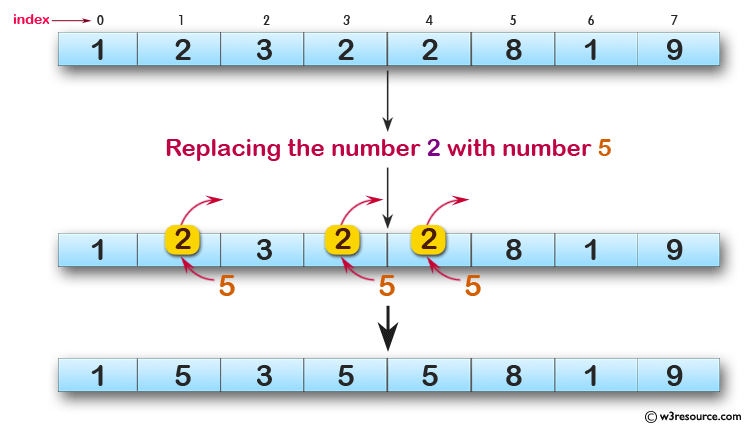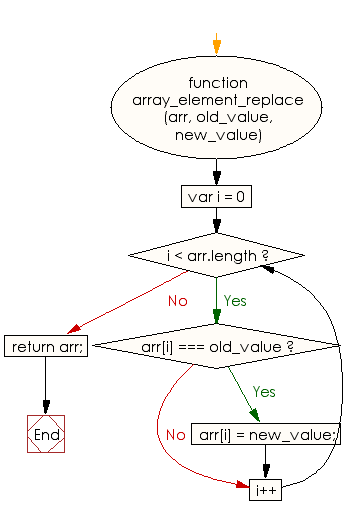# JavaScript: Replace all the numbers with a specified number of a given array of integers

## JavaScript Basic: Exercise-95 with Solution

Write a JavaScript program to replace all the numbers with a specified number of a given array of integers.

Pictorial Presentation:Sample Solution:

HTML Code:

``````<!DOCTYPE html>
<html>
<meta charset="utf-8">
<meta name="viewport" content="width=device-width">
<title>Replace all the numbers with a specified number of a given array of integers</title>
<body>

</body>
</html>
```
```

JavaScript Code:

``````function array_element_replace(arr, old_value, new_value) {
for (var i = 0; i < arr.length; i++) {
if (arr[i] === old_value) {
arr[i] = new_value;
}
}
return arr;
}
num = [1, 2, 3, 2, 2, 8, 1, 9];
console.log("Original Array: "+num);
console.log(array_element_replace(num, 2, 5));
```
```

Sample Output:

```Original Array: 1,2,3,2,2,8,1,9
[1,5,3,5,5,8,1,9]
```

Flowchart:ES6 Version:

``````function array_element_replace(arr, old_value, new_value) {
for (let i = 0; i < arr.length; i++) {
if (arr[i] === old_value) {
arr[i] = new_value;
}
}
return arr;
}
num = [1, 2, 3, 2, 2, 8, 1, 9];
console.log(`Original Array: \${num}`);
console.log(array_element_replace(num, 2, 5));
``````

Live Demo:

See the Pen javascript-basic-exercise-95 by w3resource (@w3resource) on CodePen.

What is the difficulty level of this exercise?

Test your Programming skills with w3resource's quiz.

﻿

## JavaScript: Tips of the Day

Returns the sum of the powers of all the numbers from start to end (both inclusive)

Example:

```const sumPower = (end, power = 2, start = 1) =>
Array(end + 1 - start)
.fill(0)
.map((x, i) => (i + start) ** power)
.reduce((a, b) => a + b, 0);
console.log(sumPower(10)); // 385
console.log(sumPower(10, 3)); // 3025
console.log(sumPower(10, 3, 5)); // 2925
```

Output:

```385
3025
2925
```